# Cumulative Heatmap Calculation

fast calculation of heatmap from given points.
Alternatives To Cumulative Heatmap Calculation
Gazepointheatmap40
5 years agogpl-3.0Python
Easy to use Python command line based tool to generate a gaze point heatmap from a csv file. 👁️
Csv Heatmap33
8 years ago1mitHTML
Dump1090.socket3000320
6 years ago3gpl-3.0Perl
collect dump1090 flight positions using socket30003 and save them as csv
Stravaheatmap18
6 years agoPython
Make a simple heatmap from your strava data
Geo Heatmap7
4 years ago1mitPython
Draws geographical heatmap from csv file
Cumulative Heatmap Calculation4
2 years agomitPython
fast calculation of heatmap from given points.
Muvias3
5 years agootherJavaScript
Regionalcorrelation3
3 years agoPython
Telematics Heatmap3
3 years agoJavaScript
A heatmap visualization library for sighting intensity of data at geographical points contained in generic CSV files
Csv Data Heat Map3
2 months ago1gpl-3.0Python
CSV Data to Heat Map Converter
Alternatives To Cumulative Heatmap Calculation
Select To Compare

Alternative Project Comparisons

# Cumulative-Heatmap-Calculation

Fast calculation of heatmap from given 2D points.

## Overview

This code is a follow-up project for calculating a heat map using a recursive algorithm: recursive-HeatMap-calculation. Calculating a heat map is a complex task, because since the user selects a more detailed resolution, the runtime of the calculation increases accordingly. The main difficulty in the calculation is in the sum of all the coordinates for the boundaries of each cell in the heatmap.

The recursive method has managed to result a significant improvement in the runtime of the code (see here). But, there are still modifications that can be made even better results. To calculate the heatmap, the code chm.py applied different approach from the previous project, and use Flooring Division to improve the runtime of the code:

• Step One: Define the division parameter for the points 2D space, so that we know the length of a side of each square we want to calculate.

• Step Two: We will use a loop through all the points, and calculate for each point its the row and column, using flooring division, when side is used as a denominator:and:• Step Three: We will use the row and column we have calculated as KEY in the dictionary, so that we can accumulate for each KEY the amount of points associated with it.

• Step Four: now we get heatmap dictionary, that maintain for each row and column the number of intersected point to its area.

An illustration of the HeatMap calculation process can be seen in the following plot. As you can see, each dot is added to a specific square on the map, so in the end of the process we get the cumulative result: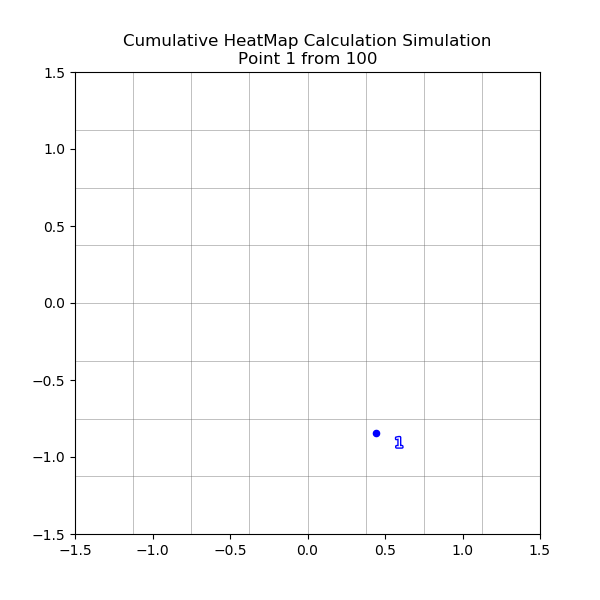This method allows a runtime of O(n) and produces only squares that overlap to the given points. This implementation allows extremely fast runtime, even for a large amount of given points. As can be seen, the runtime of the calculation using the cumulative algorithm is significantly faster, relative even to the recursive algorithm: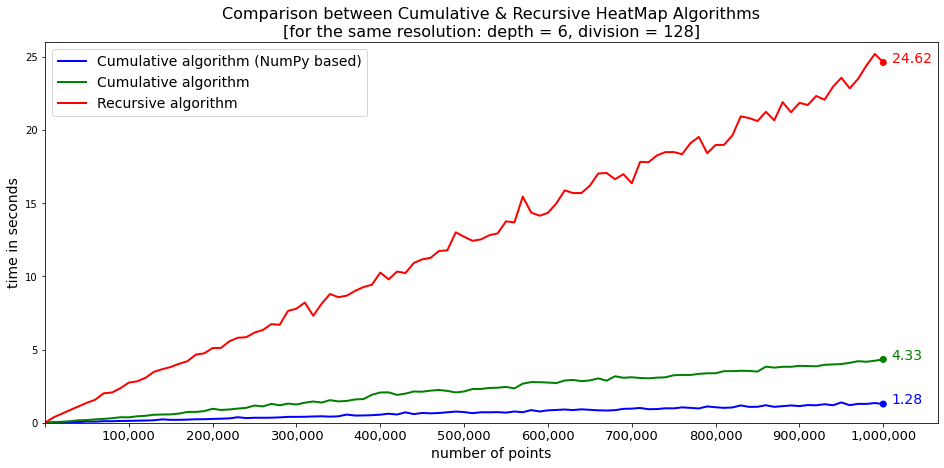The resolution of the heatmap can be adjusted using the "division" variable. This variable determines to how many parts should the points 2D space should divide. The larger the "division" variable, the higher the resolution we get. You can see a diagram describing the split into squares, by each division: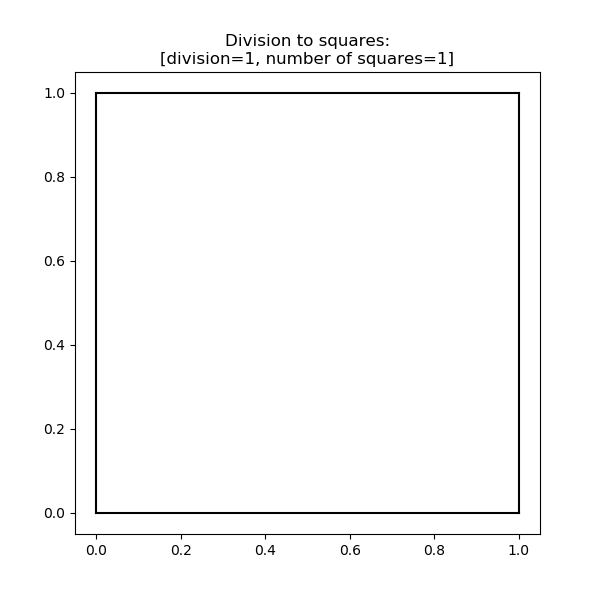As mentioned, it is important to make sure that a suitable resolution is chosen for the calculation, since different values for the "division" variable will lead to different results. A simple example based on the file Athens.kml, illustrates how different values resulted different outputs: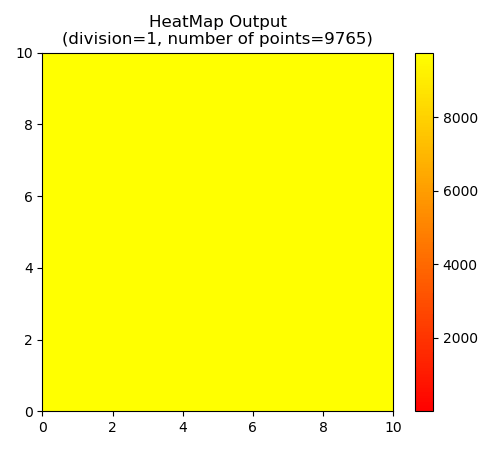The heat map calculation performed using the HeatMap object. This object receives a Python list consisting of tuples of X and Y coordinates. Also, the desired division also must be set for the object. Using the data about the coordinates, the HeatMap calculates the intersections. The object procces the coordinates to heatmap result, such as this example, based on the file data.npy, that contain 1,000,000 points!: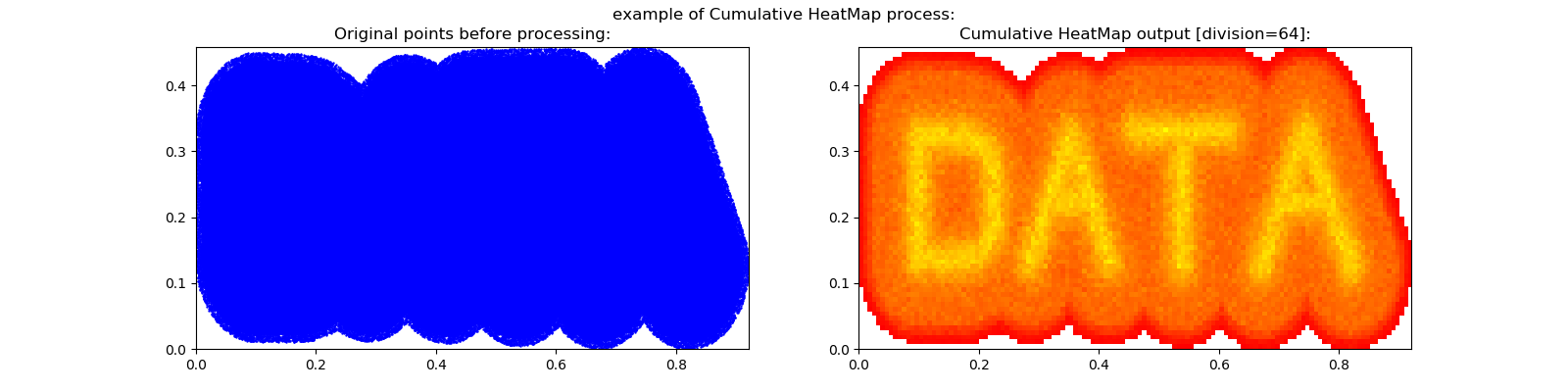The final squares can be accessed as one of the data of the object: HeatMap(xy_tpl_lst, division = 100).get_map(). The function return list of dictionaries(one for each square), each one contain two value:

• "xy": conatian the X and Y coordinate values of the square
• "count": conatian the number of the points intersect with the square

It is also possible to create a plot based on the results of the heat map using the function built into the object, as in the following example:

``````from chm import HeatMap, loadkml

hm = HeatMap(xy, division=80)
hm.plot()
``````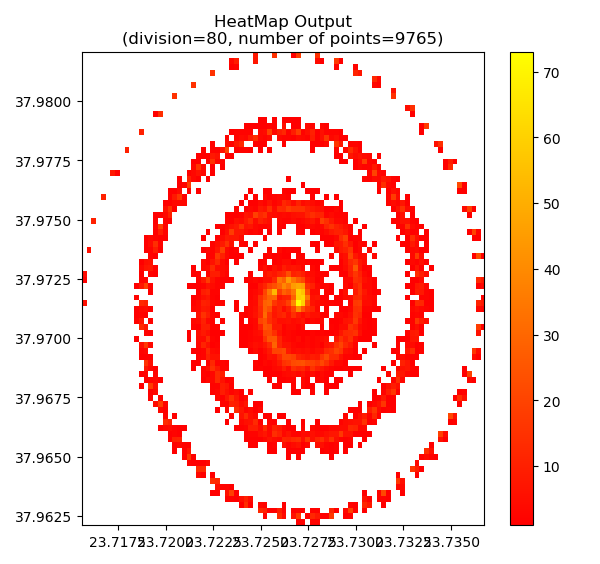In addition, the calculation results can be exported and saved as files in various formats using the save_map function of the object, as can be seen in the implementation.py file. The function allows to save the results in SHP, KML and CSV format. It should be noted that saving the results as layers is automatically set to geographic coordinate system of WGS_1984_DD.

Also, the data that saved to a KML file gets a color corresponding to the count of its intersection. Example for such output you can see here as interactive MYMAPS page. This output based on the Athens.kml example file and the output also available here: Athens_output.kml. Also, for convenience, the code contains three XY-tuple-list load functions from SHP, KML and CSV files:

• loadcsv (this function required also the X & Y fields names)

The Cumulative-Heatmap-Calculation code was originally written as a standard Python script, but now it rebuild by using Numpy implementation. This new vrsion increase the speed, over the previous version significantly (see here for runtime comparsion). The old version of the code is also available, in the following path: old version

## Libraries

The code uses the following libraries in Python:

matplotlib

shapefile

simplekml

pandas

numpy

## Application

An application of the code is attached to this page under the name:

implementation.py

the examples outputs are also attached here.

## Example for using the code

To use this code, you just need to import it as follows:

``````# import

# define division-resolution variable
divison = 5

# application
hm = HeatMap(xy, divison)

# plot
hm.plot()

# save data as csv
hm.save_map('filename','csv')
``````

When the variables displayed are:

xy: the given xy coordiantes list.

division the required divisions for the 2D space, define the output resolution.